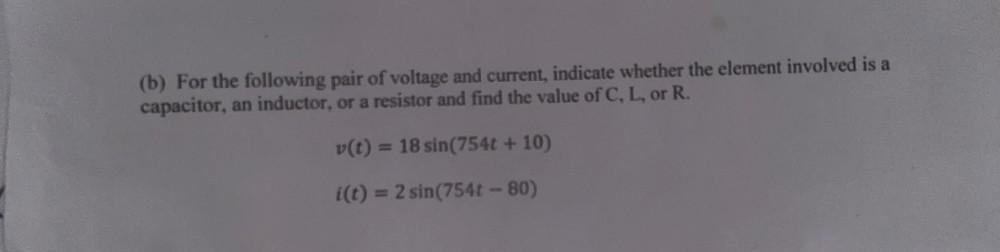Question:

# (b) For the following pair of voltage and current, indicate whether the element involved is a capacitor, an inductor, or a resis(b) For the following pair of voltage and current, indicate whether the element involved is a capacitor, an inductor, or a resistor and find the value of C, L, or R. v(t) = 18 sin(754t + 10) i(t) = 2 sin(754t-80)